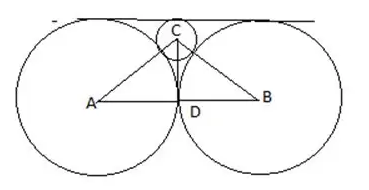# Two circles, each of radius 4 cm | Quantitative Aptitude - Geometry – Circles

## CAT 2019 - Slot 2 - Quantitative Aptitude - Circles - Question 1 - Two circles, each of radius 4 cm

Q. 1: Two circles, each of radius 4 cm, touch externally. Each of these two circles is touched externally by a third circle. If these three circles have a common tangent, then the radius of the third circle, in cm, is

1. π/3

2. 1/√2

3. √2

4. 1

See the figure,In above figure , AD=BD =4 , let radius of 3rd circle = r

So AC = 4+r, CD = 4-r

Using Pythagoras theorem in right angled triangle ADC,

(4+r)^2 = 4^2 + (4-r)^2

16+ r^2 + 8r = 16 + 16 + r^2 – 8r

16r = 16

r =1

### Past Year Question Paper & SolutionsCounselling Session
By IIM Mentor

#### Free Material Area